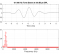## Matlab Optimization Toolbox-Constrained Optimization problemStarted by 7 years ago5 replieslatest reply 7 years ago316 views
Hi,

I have a problem on solving a constrained optimization problem which has 5 variables and 3 constraints.

my code for the objective function is:
function y = objective_function(x)
y = 225*x(1)-45*x(2)-80*x(3)-1000*x(4)-90*x(5);
end

Which I saved in an m file.

The code for my constraint equations:
function [c,ceq] = constraint_equation (x)
c = [];
ceq = x(1)-4930-0.7548*x(3);
x(3)-((27)*(375-((217.5+0.039*x(3))/((548.8/x(5))+1)))*(1-exp(-0.005556*x(4))));
[x(2)-45*(557.5+0.039*x(3)-((217.5+0.039*x(3))/((548.8/x(5))+1)))];
end

Which I saved in another m file.
The initial values were given as follow
x(1)=6000
x(2)=9000
x(3)=1200
x(4)=100
x(5)=25

After plugging them all in the optimization toolbox, I used the 'fmincon' solver and SQP as the algorithm. After running the system, I obtained a negative answer which is totally wrong. Really help anyone of you can help me on this!
[ - ]Out of curiosity, how do you know the answer is wrong?  Please discuss your rationale for reaching this conclusion.

[ - ]If I solve the problem by using the Newton Raphson method, the objective value is y=769036.96.

However, when I use the toolbox, I get a negative answer. Also, the question asked to maximize profit y. Sorry, its my bad for not clearly stating the problem.

I've also tried to write the code by using the 'fmincon' solver.

options = optimoptions('fmincon','Display','iter','Algorithm','sqp');

fun = @(x)-(225*x(1)-45*1000*x(4)-90*x(3)-1000*x(4)-80*x(5));

A = [];

b = [];

Aeq = [1,0,0,0,-0.7548];

beq = ;

lb = [];

ub = [];

nonlcon = @constraint_equation;

x0 = [12013.10423,189391.9536,-3.4,450,9384.08086];

x = fmincon(fun,x0,A,b,Aeq,beq,lb,ub,nonlcon,options);

[x,fval] = fmincon(fun,x0,A,b,Aeq,beq,lb,ub,nonlcon,options)

And the result is:

x =

1.0e+15 *

0.0000    0.0000   -0.0172   -8.7719    0.0000

fval =

-4.0351e+20

Really need much help here. Thank you!

[ - ]Also, please ignore the above constraint equation. Here's the right one.

function [c,ceq] = constraint_equation (x)

c = [];

ceq = x(1)-4930-0.7548*x(5);x(5)-((27)*(375-((217.5+0.039*x(5))/((548.8/x(3))+1)))*(1-exp(-0.005556*x(4))));[x(2)-45*(557.5+0.039*x(5)-((217.5+0.039*x(5))/((548.8/x(3))+1)))];

end

[ - ]It looks like you've taken into account the change in sign necessary to change the maximization problem into a minimization problem that is suitable for the FMINCON function.  However, why did your objective function change?

You originally posted:

y = 225*x(1)-45*x(2)-80*x(3)-1000*x(4)-90*x(5);

Then you posted:

fun = @(x)-(225*x(1)-45*1000*x(4)-90*x(3)-1000*x(4)-80*x(5));

[ - ]